• ## matlab的parcorr函数

千次阅读 2016-02-24 15:54:49
零均值的平稳时间序列： 样本自协方差 样本自相关系数函数： 偏相关函数：从中解出的一串 程序如图所示clear ; A = [1 2 3 4] ; n = length(A) ; [ACF,lags,bounds] = autocorr(A,n-1) ; % ACF
参考文章http://wenku.baidu.com/view/32b8f843fc4ffe473268ab2a.html
零均值的平稳时间序列：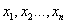样本自协方差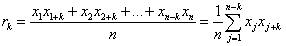样本自相关系数函数：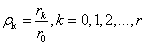偏相关函数：从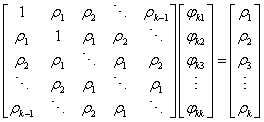中解出的一串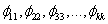程序如图所示

clear ;
A = [1 2 3 4] ;
n = length(A) ;
[ACF,lags,bounds] = autocorr(A,n-1) ;
% ACF = [1,0.2500,-0.3000,-0.4500]
%构造矩阵[1     0.25    -0.3
%         0.25  1       0.25
%         -0.3  0.25    1    ]
B = [1 0.25 -0.3 ; 0.25 1 0.25 ; -0.3  0.25 1 ] ;
%构造向量[0.25;-0.3;-0.45]
C = ACF(2:4)' ;
%保存 x11 ,x22 ,x33
x = zeros(n-1,1) ;

%计算x11
% [x11] = [0.25] ====>x11 = 0.25
x(1,1) = C(1) ;

%计算x22
%[1 0.25 ; 0.25 1][x21 ; x22] = [0.25;-0.3]
temp =  B(1:2,1:2) \ C(1:2) ;
x(2,1) = temp(end) ;

%计算x33
%[1 0.25 -0.3 ; 0.25 1 0.25 ; -0.3 0.25 1][x11;x22;x33] = [0.25;-0.3;-0.45]
temp =  B \ C ;
x(3,1) = temp(end) ;
x = [1;x] ;
subplot(2,1,1) ;
plot([0:n-1],x',[0:n-1],0,'-k',[0:n-1],x','*') ;
title('自己求偏相关') ;

subplot(2,1,2) ;
[PartialACF,lags,bounds] = parcorr(A,n-1) ;
plot(lags,PartialACF,lags,0,'-k',lags,PartialACF,'*') ;
title('parcorr求偏相关') ;

得到的图像如图所示：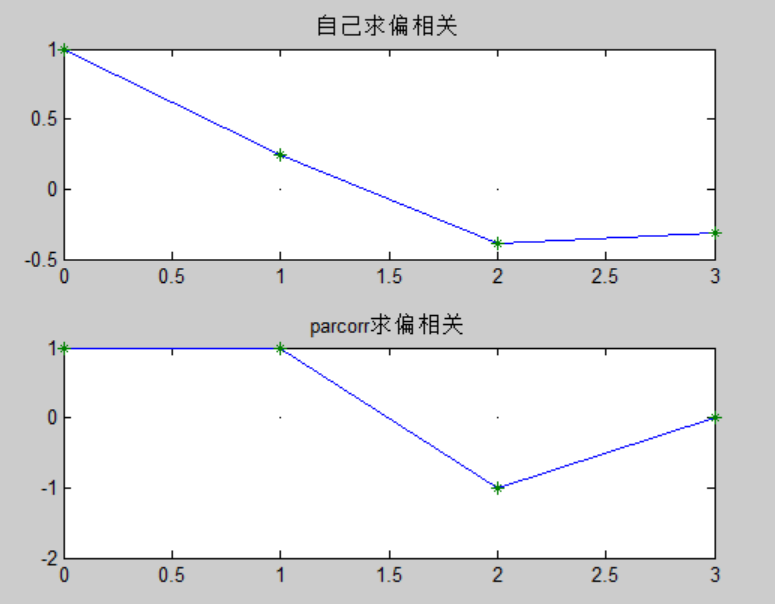得到的图像不怎么相似，以为自己的错了，然后在上面参考文章里找到了求偏相关函数的程序

clear ;
A = [1 2 3 4] ;
n = length(A) ;

%[ACF,lags,bounds] = autocorr(A,n-1)
% ACF = 1 0.25 -0.3 -0.45

%求自相关函数
A = A - mean(A) ;
rc = 0 ;
for k = 1 : n
rc = rc + A(k)*A(k)/n ;
end

for k = 1 : n-1
x = 0 ;
for j = 1 : n-k
x = x + A(j)*A(j+k)/n ;
end
r(k) = x ;
p(k) = r(k) / rc ;
end

x = 1 : n-1 ;
figure;plot(x,p,x,0,'-k',x,p,'*') ;
title('自相关函数') ;

%求偏相关函数m(k,k)
m(1,1) = p(1) ;
for k = 1 : n-2
x = 0 ;
y = 0 ;
for j = 1 : k
x = x + p(k+1-j) * m(k,j) ;
y = y + p(j) * m(k,j) ;
end

m(k+1,k+1) = (p(k+1)-x) / (1-y) ;

for j=1 : k
m(k+1,j) = m(k,j) - m(k+1,k+1)*m(k,k-j+1) ;
end
end

result = [1,(diag(m))'] ;
plot([0:n-1],result,[0:n-1],0,'-k',[0:n-1],result,'*') ;
axis([0 3 -0.5 1]) ;
title('验证自己求偏相关正确') ;


为了方便对比，我把图像集成到了一起，如图所示：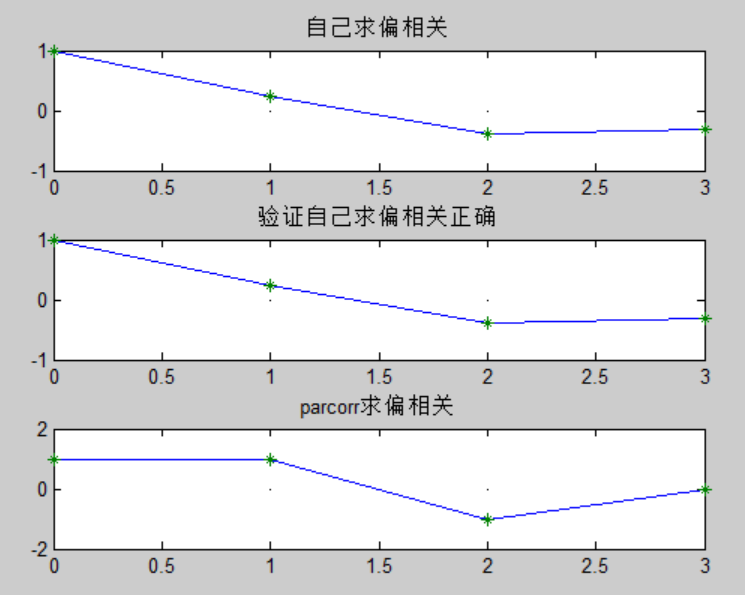。

为什么自己写的偏相关程序与parcorr函数不一样呢，我也不知道！！！
但是我们可以在序列比较大的情况下再对比一下。

clear ;
z = [15600 8960 10400 10600 10800 9880 9850 10900 8810 9960 ...
12200 7510 8640 6380 6810 8820 14400 7440 7240 6430 11000 ...
7340 9260 5290 9130 7480 6980 9650 7260 8750 9900 7310 9040 ...
7310 8850 7840 10700 6190 9610 7580 9990 6150 8250 6030 8980 ...
6180 9630 9490 2340 11100 5090 10900 6490 12600 6640 7430 6760 ...
10000 9300] ;

m = mean(z) ;
for k = 1 : 59
X(k) = z(k) - m ;
end

%求自相关函数
rc = 0 ;
for k = 1 : 59
rc = rc + 1/59*X(k)*X(k) ;
end

for k=1:15
x=0;
for j=1: 59-k
x = x + 1/59*X(j)*X(j+k) ;
end

r(k) = x ;
p(k) = r(k) / rc ;
end

x = 1 : 15 ;
figure;plot(x,p,x,0,'-k',x,p,'*') ;
title('自相关函数');

%求偏相关函数m(k,k)
m(1,1) = p(1) ;
for k = 1 : 14
x = 0 ;
y = 0 ;
for j = 1 : k
x = x + p(k+1-j) * m(k,j) ;
y = y + p(j) * m(k,j) ;
end

m(k+1,k+1) = (p(k+1)-x) / (1-y) ;

for j = 1 : k
m(k+1,j) = m(k,j) - m(k+1,k+1) * m(k,k-j+1) ;
end
end

result = [1,(diag(m))'] ;
x = 0 : 15 ;
figure;subplot(2,1,1) ;
plot(x,result,x,0,'-k',x,result,'*') ;
title('自己求偏相关函数') ;

subplot(2,1,2) ;
[PartialACF,lags,bounds] = parcorr(z,15) ;
plot(lags,PartialACF,lags,0,'-k',lags,PartialACF,'*') ;
title('parcorr求偏相关') ;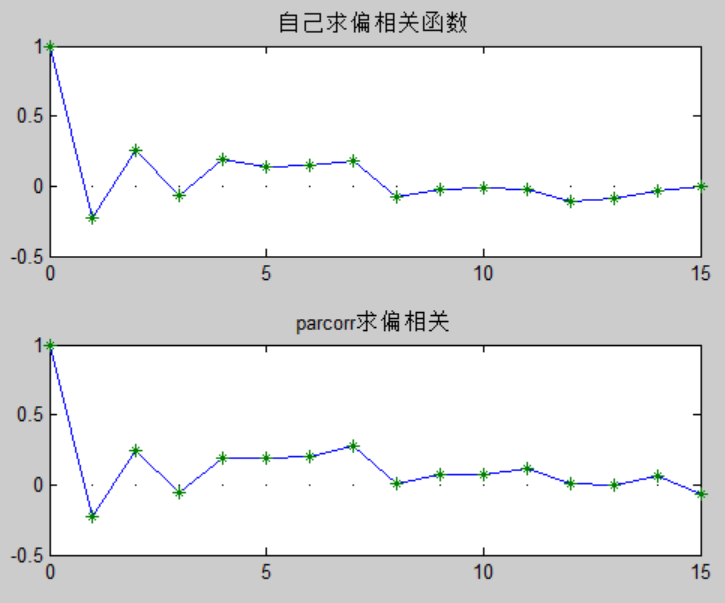可以看出来，虽然每个具体值还存在偏差，但是整体趋势还是一致的，从而可以说明我们写的偏相关函数是正确的。

既然偏相关函数搞清楚了，我们就了解一下ARMA模型识别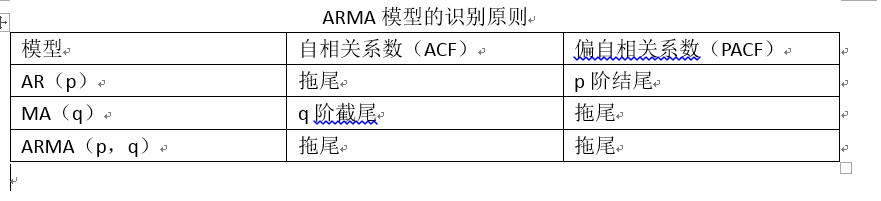举个例子：

clear ;
z = [15600 8960 10400 10600 10800 9880 9850 10900 8810 9960 ...
12200 7510 8640 6380 6810 8820 14400 7440 7240 6430 11000 ...
7340 9260 5290 9130 7480 6980 9650 7260 8750 9900 7310 9040 ...
7310 8850 7840 10700 6190 9610 7580 9990 6150 8250 6030 8980 ...
6180 9630 9490 2340 11100 5090 10900 6490 12600 6640 7430 6760 ...
10000 9300] ;
figure ;parcorr(z,length(z)-1) ;

figure ;autocorr(z,length(z)-1) ;

自相关函数如图所示，3阶结尾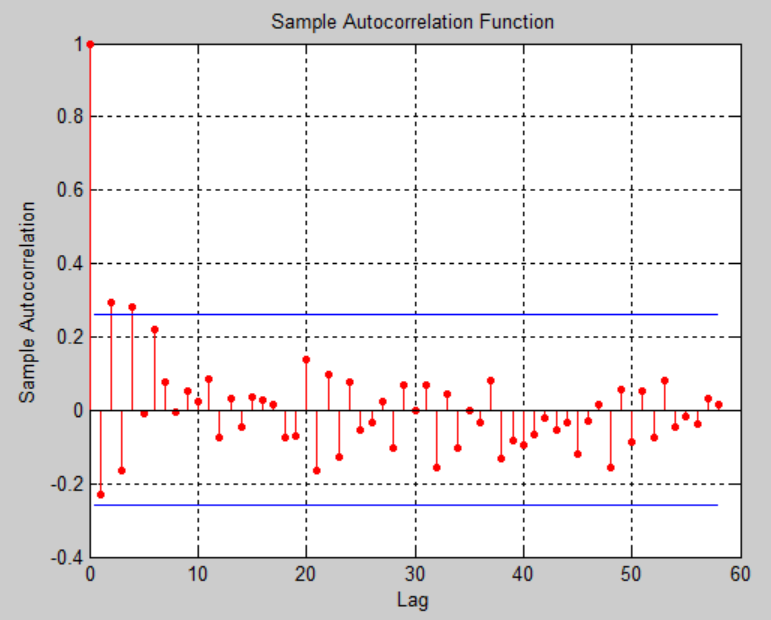偏相关函数如图所示，具有拖尾现象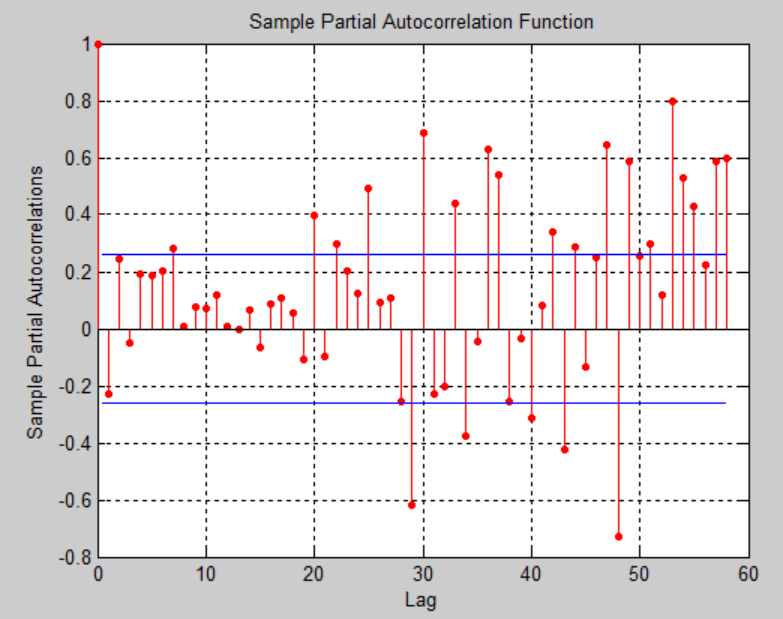，所以上面的序列可以判断为MA（3）模型
展开全文ARMA
• 相关函数的程序 1.1 通用信息查询(General information) demo 演示程序 help 在线帮助指令 helpbrowser 超文本文档帮助信息 helpdesk 超文本文档帮助信息 helpwin 打开在线帮助窗 info MATLAB 和MathWorks ...
• 信号相关函数的概念 信号相关分析 (Cross-correlation) 是一种分析两个信号之间或一个信号自身的时间依存关系和相似程度的方法 ρxy(τ)=∫−∞+∞x(t)y(t−τ)dt[∫−∞+∞x2(t)dt∫−∞+∞y2(t)dt]1/2\rho_{xy}...
信号的时差域相关分析

华中科技大学《数字信号分析理论实践》第五单元 信号的时差域分析 学习总结记录

信号相关函数的概念

信号相关分析 (Cross-correlation) 是一种分析两个信号之间或一个信号自身的时间依存关系和相似程度的方法

$\rho_{xy}(\tau)=\frac{\int_{-\infty}^{+\infty}x(t)y(t-\tau)dt}{[{\int_{-\infty}^{+\infty}x^2(t)dt\int_{-\infty}^{+\infty}y^2(t)dt]}^{1/2}}$

变量相关的概念

统计学中用相关系数来描述变量 $x,y$ 之间的相关性，它是两随机变量之积的数学期望，表征了 $x,y$ 间的关联程度

$\rho_{xy}=\frac{c_{xy}}{\sigma_x\sigma_y}=\frac{E[(x-\mu_x)(y-\mu_y)]}{\{E[(x-\mu_x)^2]E[(y-\mu_y)^2]\}^{1/2}}$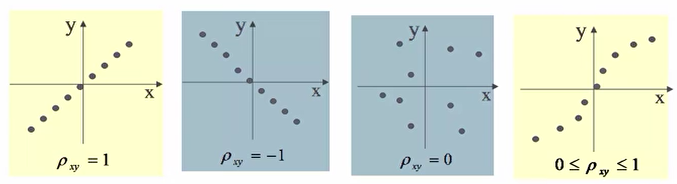波形相关的概念（相关函数）

如果所研究的变量 $x,y$ 是与时间有关的函数，即 $x(t)$ 与 $y(t)$ ，则其相关系数也是随其相对时刻变化的函数
这时可以引入一个与相对时间差 $\tau$ 有关的量，称为函数的相关系数或相关函数，有

$\rho_{xy}(\tau)=\frac{\int_{-\infty}^{+\infty}x(t)y(t-\tau)dt}{[{\int_{-\infty}^{+\infty}x^2(t)dt\int_{-\infty}^{+\infty}y^2(t)dt]}^{1/2}}$

相关函数反映了两个信号在时移的相关性
工程上，人们关心的是信号不同时刻的相似程度，但不太关心其具体值，这时相关函数可简化为

$R_{xy}(\tau)=\int_{-\infty}^{+\infty}x(t)y(t+\tau)dt$
[FileName,PathName] = uigetfile('*.mp3','Select mp3 File');
abc = fullfile(PathName,FileName);
[y,Fs] = audioread(abc);
figure
subplot(211);
plot(y);
s = xcorr(y,'unbiased');
subplot(212);
plot(s);


图形说明，找不到回波音频，随便找了一个 MP3 文件扔进去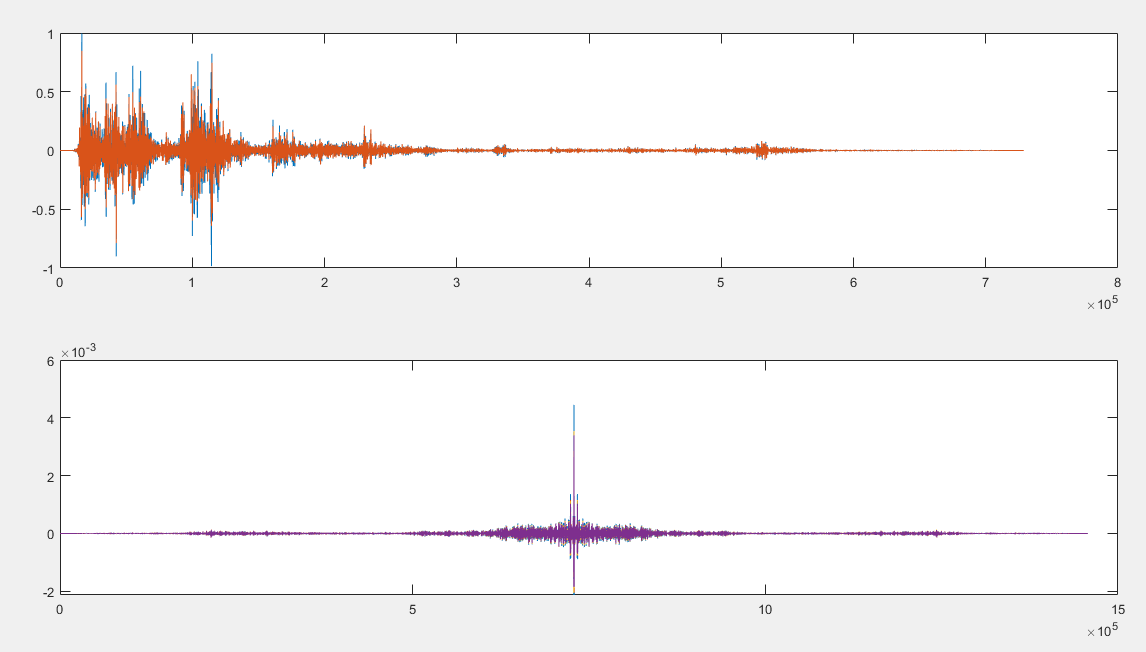相关函数的性质

相关函数描述了信号间或信号自身不同时刻的相似程度

自相关函数是偶函数，$R_x(\tau)=R_x(-\tau)$
当 $\tau = 0$ 时，自相关函数具有最大值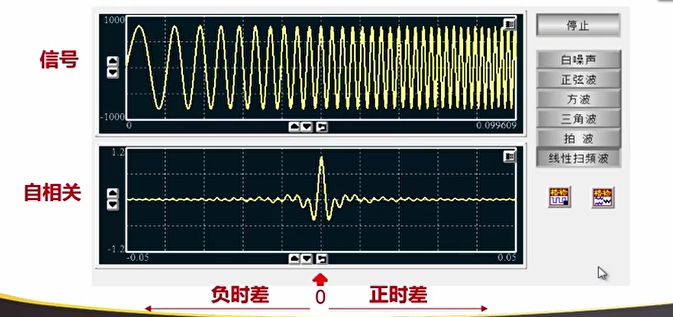N = 1024;
T = 0.2;
t = linspace(0,T,N);
n = randn(1,N);
subplot(211)
plot(t,n);
r = xcorr(n,'unbiased');
t1 = linspace(-T,T,2*N-1);
subplot(212);
plot(t1,r);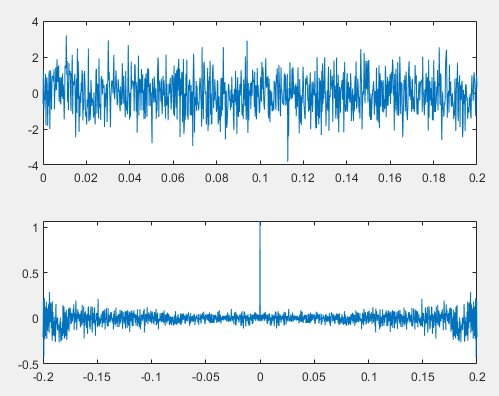周期信号的自相关函数仍然是同频率的周期信号，但不保留原信号的相位信息
随机噪声信号的自相关函数将随 $\tau$ 的增大快速衰减，相关滤波
两周期信号的互相关函数仍然是同频率的周期信号，且保留原来信号的相位信息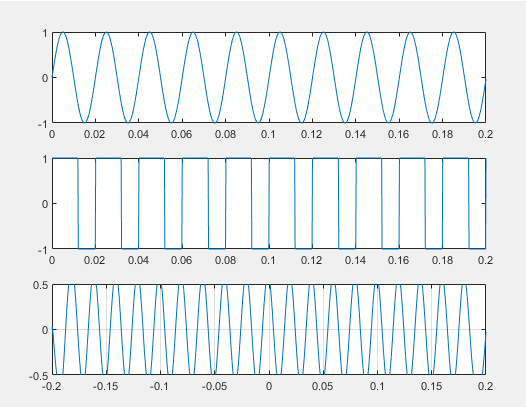N = 1024;
T = 0.2;
t = linspace(0,T,N);
s1 = sin(2*3.14*50*t);
subplot(311)
plot(t,s1);
s2 = square(2*pi*50*t,60);
subplot(312)
plot(t,s2);
r = xcorr(s1,s2,'unbiased');
t1 = linspace(-T,T,2*N-1);
subplot(313);
plot(t1,r);
ylim([-0.5,0.5])
grid on


两个非同频率的周期信号互不相关，相乘积分等于0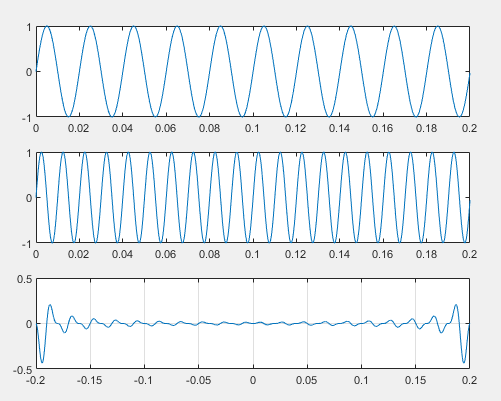N = 1024;
T = 0.2;
t = linspace(0,T,N);
s1 = sin(2*3.14*50*t);
subplot(311)
plot(t,s1);
s2 = sin(2*3.14*100*t);
subplot(312)
plot(t,s2);
r = xcorr(s1,s2,'unbiased');
t1 = linspace(-T,T,2*N-1);
subplot(313);
plot(t1,r);
ylim([-0.5,0.5])
grid on


建议去看原视频，有随着相位改变信号移动的图

相关函数的数学计算方法

相关函数公式

$R_{xy}(\tau)=\int_{-\infty}^{+\infty}x(t)y(t+\tau)dt$

数字信号离散计算公式

$R_{xy}(k)=\sum_{0}^{N-1}x(n)y(n+k)k=0,1,\dots,N-1$

双重循环计算量大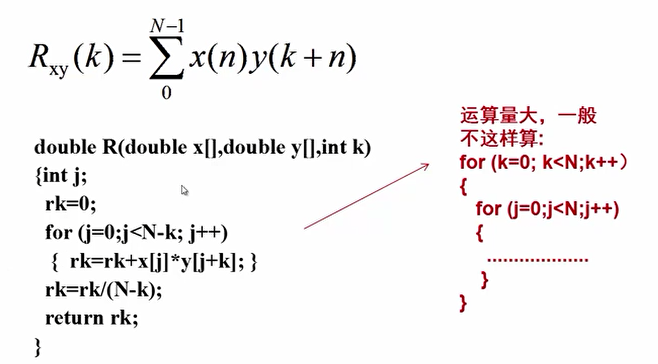快速算法：频域相乘等于时域卷积
$x(n)\overset{FFT}{\rightarrow}X(k)$
$y(n)\overset{FFT}{\rightarrow}Y(k)$
$\Rightarrow R(k)=X(k)\overline{Y}(k)\Rightarrow R(k)\overset{IFFT}{\rightarrow}r(n)$

N = 1024;
T = 0.2;
t = linspace(0,T,N);
y = sin(2*3.14*50*t);
subplot(311)
plot(t,y);
s1 = xcorr(y,'unbiased'); % 加上无偏的参数
s2 = xcorr(y);
t1 = linspace(-T,T,2*N-1);
subplot(312)
plot(t1,s1);
subplot(313)
plot(t1,s2);


FFT 计算引入周期延拓问题，为了避免重叠失真，补等宽的零，导致另外一个问题，相关系数越来越小，以零值为中心向两边衰减，因此要加上无偏来修正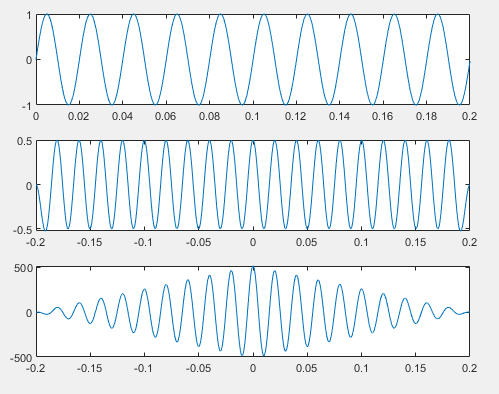相关分析应用——声波传播速度测量，雷达测距，相关滤波
N = 1024;
T = 0.2;
t = linspace(0,T,N);
s = sin(2*3.14*50*t);
subplot(411)
plot(t,s);
n = randn(1,N);
subplot(412)
plot(t,n);
y = s + n;
subplot(413);
plot(t,y);
r = xcorr(y,'unbiased'); % 加上无偏的参数
t1 = linspace(-T,T,2*N-1);
subplot(414);
plot(t1,r);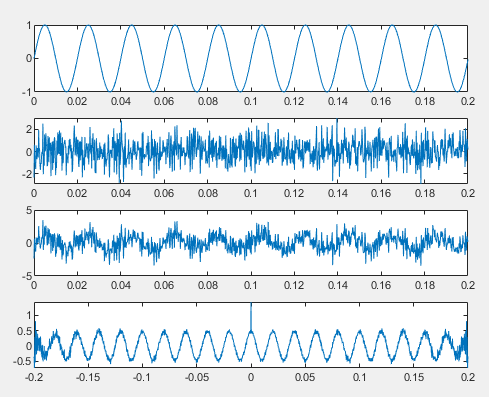找老师上课画的图，时间差是回波的时间差，计算两个峰点的时间，乘以声速得到距离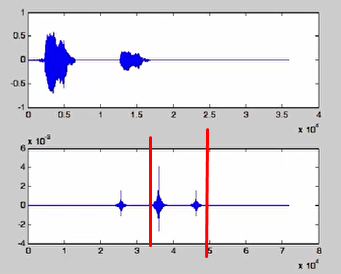展开全文• 偏相关图 %误差值的正态性的检验 figure,hist(errors);%频数直方图 figure,normplot(errors);%Q-Q图 [muhat,sigmahat,muci,sigmaci]=normfit(errors);%参数估计 均值,方差,均值的0.95置信区间,方差的0.95置信区间 ...

频数直方图
Q-Q图
参数估计
假设检验
自相关图
偏相关图

%误差值的正态性的检验
figure,hist(errors);%频数直方图
figure,normplot(errors);%Q-Q图
[muhat,sigmahat,muci,sigmaci]=normfit(errors);%参数估计 均值,方差,均值的0.95置信区间,方差的0.95置信区间
[h1,sig,ci]= ttest(errors,muhat);%假设检验
figure, ploterrcorr(errors);%绘制误差的自相关图
figure, parcorr(errors);%绘制偏相关图


展开全文• matlab随机数的产生： 二项分布随机数的产生： clear all; r=binornd(6,0.8) R=binornd(6,0.8,4,5) %产生一个4* 5的矩阵 泊松分布： clear all; r=poissrnd(6) %泊松分布 R=poissrnd(6,3,3) %产生一个3* 3的矩阵 ...
matlab随机数的产生：
二项分布随机数的产生：
clear all;
r=binornd(6,0.8)
R=binornd(6,0.8,4,5)   %产生一个4* 5的矩阵
泊松分布：
clear all;
r=poissrnd(6)       %泊松分布
R=poissrnd(6,3,3)     %产生一个3* 3的矩阵
指数分布：
clear all;
r=exprnd(5)        %指数分布
R=exprnd(5,5,5)     %产生一个5* 5的矩阵
连续型均匀分布：
clear all;
r=unifrnd(1,5)              %均匀分布   1到5之间
R1=unifrnd(1,5,3,3)         %产生一个3* 3的矩阵
R2=unifrnd(1,5,[3 3])
离散型均匀分布：
clear all;
r=unidrnd(6)           %离散型均匀分布
R1=unidrnd(6,3,3)       %产生一个3* 3的矩阵
R2=unidrnd(6,[3 3])
正态分布：  应用最广  非常重要
clear all;
r=normrnd(0,1)
R1=normrnd(0,1,[3 4])   %产生一个3* 4的矩阵   均值为0   标准差为1
R2=normrnd(2,4,[3 4])
matlab求平均值和中位数
算数平均值：
clear all;
A=[1 2 3 4;4 4 4 4]
m1=mean(A)              %对列元素求算术平均值
m2=mean(A,2)            %对行元素求算术平均值
忽略非数（有的元素不是数值）计算算术平均值：
clear all;
A=[1 4 nan 5;6 nan 7 nan]
m1=mean(A)                  %有nan就是nan
m2=nanmean(A)               %对列元素求算术平均值
m3=nanmean(A,2)             %对行元素求算术平均值
几何平均数：
clear all;
A1=[6 2 2 4]
m1=geomean(A1)      %向量的几何平均数
A2=[1 4 5;7 7 8]
m2=geomean(A2)      %列元素的几何平均数
m3=geomean(A2,2)    %行元素的几何平均数
调和平均数：
clear all;
A1=[1 2 3 4]
m1=harmmean(A1)      %向量的调和平均数
A2=[1 2 2;2 2 4]
m2=harmmean(A2)      %列元素的调和平均数
m3=harmmean(A2,2)    %行元素的调和平均数
中位数：
clear all;
A1=[1 2 3 4]
m1=median(A1)            %向量的中位数
A2=[1 6 3;2 6 4;4 6 8]
m2=median(A2)            %列元素的中位数
m3=median(A2,2)          %行元素的中位数
nan   中位数：
clear all;
A1=[1 2 nan 1 nan 3 4]
m1=nanmedian(A1)               %向量的中位数
A2=[1 2 nan;2 nan 4;4 1 8]
m2=median(A2)%只要有非数值类型都是非数值
m3=nanmedian(A2)               %列元素忽略NaN的中位数
m4=nanmedian(A2,2)             %行元素忽略NaN的中位数
常见分布的概率密度函数：
二项分布的密度函数：
clear all;
x=1:20;
y=binopdf(x,200,0.06);
figure;
plot(x,y,‘r*’);
title(‘二项分布（n=200，p=0.06）’);
泊松分布的密度函数：
clear all;
x=1:20;
y=poisspdf(x,20);       %泊松分布
figure;
plot(x,y,‘r+’);
title(‘泊松分布’);
几何分布：
clear all;
x=1:10;
y=geopdf(x,0.4);        %几何分布
figure;
plot(x,y,‘rx’);
title(‘几何分布’);
均匀分布（离散）：
clear all;
n=10;
x=1:n;
y=unidpdf(x,n);             %均匀分布（离散）
figure;
plot(x,y,‘ro’);
title(‘均匀分布（离散）’);
均匀分布（连续）：
clear all;
x=-2:0.1:15;
y=unifpdf(x,0,6);           %均匀分布（连续）   0到6之间
figure;
plot(x,y,‘r:’);
title(‘均匀分布（连续）’);
指数分布：
clear all;
x=0:0.1:10;
y=exppdf(x,2);      %指数分布
figure;
plot(x,y,‘r:’);
title(‘指数分布’);
正态分布：
clear all;
x=-5:0.1:5;
y1=normpdf(x,0,1);          %标准正态分布
y2=normpdf(x,3,3);          %非标准正态分布
figure;
plot(x,y1,x,y2,’:’);
legend(‘标准正态分布’,‘非标准正态分布’);
x1=-5:0.1:5;
y3=normpdf(x1,3,1);         %SIGMA=1
y4=normpdf(x1,3,2);         %SIGMA=2
y5=normpdf(x1,3,3);         %SIGMA=3
figure;
plot(x1,y3,‘r-’,x1,y4,‘b:’,x1,y5,‘k–’);
legend(‘SIGMA=1’,‘SIGMA=2’,‘SIGMA=3’);
y6=normpdf(x1,0,2);                   %MU=0
y7=normpdf(x1,2,2);                   %MU=2
y8=normpdf(x1,4,2);                   %MU=4
figure;
plot(x1,y6,‘r-’,x1,y7,‘b:’,x1,y8,‘k–’);
legend(‘MU=0’,‘MU=2’,‘MU=4’);
三大抽样分布的概率密度函数：
卡方分布：
clear all;
x=0:0.1:15;
y1=chi2pdf(x,2);    %卡方分布n=2
y2=chi2pdf(x,3);    %卡方分布n=3
figure;
hold on;
plot(x,y1);
plot(x,y2,’:’);
legend(‘n=2’,‘n=3’);
title(‘卡方分布’);
%%  t分布
clear all;
x=-5:0.1:5;
y1=tpdf(x,2);            %t分布（n=2）
y2=tpdf(x,10);           %t分布（n=10）
figure;
plot(x,y1,‘r:’,x,y2,‘b-’);
legend(‘n=2’,‘n=10’);
title(‘t分布’);
%% F分布
clear all;
x=0.1:0.1:5;
y=fpdf(x,2,5);             %F分布
figure;
plot(x,y,‘r:’);
title(‘F分布（m=2,n=5）’);
常见分布的期望和方差：
二项分布的期望和方差：
clear all;
n1=100;
p1=0.3;
[m1,v1]=binostat(n1,p1) %1000.3   1000.3*0.7
clear all;
a1=1;
b1=5;
[m1,v1]=unifstat(a1,b1)
正态分布的期望和方差：
clear all;
n1=2;
n2=3;
[m1,v1]=normstat(n1,n2)
指数分布的期望和方差：
clear all;
mu1=2;
[m1,v1]=expstat(mu1)
t分布的期望和方差：
clear all;
n1=4;
[m1,v1]=tstat(n1)
F分布的期望和方差：
clear all;
n1=6;
n2=8;
[m1,v1]=fstat(n1,n2)
排序：
clear all;
X=[1 3 4;8 3 5;2 7 4]
y1=sort(X)                  %按列由小到大排序
y2=sort(X,2)                %按行由小到大排序
y3=sort(X,1,‘descend’)      %按列由大到小排序
按行排序：
clear all;
X=[1 3 8;8 8 5;82 7 4]
y1=sortrows(X)              %按行排序
y2=sort(X,2)                %按指定的列排序
求最大值和最小值的差：
clear all;
X1=[1 3 4 10 3 5]
y1=range(X1)                 %向量
X2=[1 3 5;2 4 6;8 4 3]
y2=range(X2)                 %矩阵
y3=range(X2,2)
求最大值和最小值：
clear all;
X1=[2 5 4 12 3 15]
y1=minmax(X1)                 %向量
X2=[1 3 5;2 4 6;8 4 3]
y2=minmax(X2)                 %矩阵
方差：
clear all;
A=rand(1,8)         %向量
y=var(A)
B=rand(3,5)         %矩阵
Y1=var(B)
标准差：
clear all;
A=[7 3 7 1;1 7 3 2;7 1 7 3]
y1=std(A)
y2=std(A,0)
y3=std(A,1)
y4=std(A,1,2)
区间参数估计： 均匀分布
clear all;
X=unifrnd(2,8,20,3)
[a,b,aci,bci]=unifit(X,0.05)%置信度0.05%
指数分布的参数估计：
clear all;
X=exprnd(5,20,4)
[parmhat1,parmci1]=expfit(X,0.05)     %置信度95%
[parmhat2,parmci2]=expfit(X,0.01)
beta分布的参数估计：
clear all;
X=betarnd(7,5,100,1);
[phat,pci]=betafit(X,0.02)
正态分布的参数估计：
clear all;
X=normrnd(10,2,20,3)
[mu1,sigma1,muci1,sigmaci1]=normfit(X,0.05)
[mu2,sigma2,muci2,sigmaci2]=normfit(X,0.01)
假设检验：
方差已知时正态总体均值的假设检验
如果已知方差和均值：
clear all;
X=[490 513 514 513 511 499 515 512 491];
[H1,P1,CI1,STATS1]=ztest(X,500,10,0.05,0)   %显著性水平ALPHA=0.05   期望是500  方差是10
[H2,P2,CI2,STATS2]=ztest(X,500,10,0.1,0)    %ALPHA=0.1       H2=1  拒绝原来假设
方差和均值未知时：
clear all;
X=[300 324 305 290 295 291 310 315];
[H,P,CI,STATS]=ttest(X,305,0.05,-1)   %ALPHA=0.05    H=0可以接受原假设
方差未知但是相等时：
clear all;
X=[302 304 305 310 320 299 298 301 315 313];
Y=[305 314 320 315 313 308 318 325 301 312];
[H,P,CI,STATS]=ttest2(X,Y,0.05,-1)            %ALPHA=0.05
分布一致性检验：
clear all;
X=[3.2 7.0 2.8 4.5 7.5 2.3 5.8 1.4];
Y=[2.3 5.6 9.2 1.2 5.9 3.4 2.8 8.4 1.5 3.2];
[P,H,STATS]=ranksum(X,Y,0.05)                   %ALPHA=0.05


展开全文• 通过理论推导证明了信号功率波形自相关函数中包含一个脉冲，利用该脉冲位置可以估计信道色散的大小。在此基础上，被称为用于相干接收系统的色散监测算法。建造了28GBaud的分立非归零零四相相移键控（PDM-NRZ-QPSK...
• 本文在Mizar系统下讨论了函数偏微分、高阶微分理论及相关性质,实现了矢量场、梯度、散度、旋度的Mizar形式,针对不同的数学问题给出了相应的算法和严格的证明,且通过了系统验证。所获结果被收录在Mizar数据库中,并...
• 证明了由该双曲型方程描述的系统不仅在D.Salamon意义下适定的,而且在G.Weiss意义下是正则的,并且证明相关的直接反馈算子为0.本文证明了Rn中三个平行耦合波方程的精确能控性和一类带有Neumann边界控制和观测的双曲型...
• , 第6章：数据分析第7章：符号数学计算, 第8章：概率统计第9章：数学建模函数, 第10章：经典智能算法, （3）第11～19 章为工程应用部分，讲解微分方程、优化、图像处理、信号处理、, 小波分析、神经网络等工具箱，...
• MATLAB环境下的正弦信号及高斯白噪声仿真程序说明 一信号的产生及时域...二信号频谱及白噪声功率谱的求解与观察 1对原正弦信号直接进行 FFT得出其频谱 2求白噪声的自相关函数随机序列自相关函数的无估计公式为 1N m 1
• MATLAB应用部分包括信号处理应用、图像处理应用、小波分析应用和微分方程应用等内容。 本书结构严谨、重点突出、条理清晰，既可以作为大中专院校相关专业以及社会有关培训班的教材，也适合广大MATLAB读者自学使用
• 1.1 MATLAB相关简介 1.1.1 MATLAB发展历程 1.1.2 MATLAB软件的特点 1.1.3 MATLAB组成 1.2 MATLAB操作界面 1.2.1 MATLAB主窗口 1.2.2 MATLAB命令窗口 1.2.3 MATLAB工作窗口 1.2.4 当前目录窗口和搜索路径 1.2.5 命令...
• 第26章 微分方程数值解有关函数介绍 第27章 利用图形用户界面（GUI）求解微分方程的一般过程 第28章 几种常见的微分方程数值求解问题 第29章 应用模式 第四篇 样条工具箱 第30章 样条工具箱及样条曲线简介 第...
• 12.3数学模型的MATLAB相关函数 12.3.1传递模型的函数 12.3.2零极点模型函数 12.3.3状态空间模型函数 第13章径向基网络算法分析与实现 13.1径向基网络模型 13.2径向基网络的学习算法 13.3广义回归神经网络 ...
• 本课件系统讲解数值方法，主要内容包括误差的概念、非线性方程求根方法、线性方程组求解、矩阵的特征值与特征向量的计算、插值、曲线拟合与函数逼近、数值积分方法、常微分方程求解、微分方程求解等。包含丰富的...
• 本课件系统讲解数值方法，主要内容包括误差的概念、非线性方程求根方法、线性方程组求解、矩阵的特征值与特征向量的计算、插值、曲线拟合与函数逼近、数值积分方法、常微分方程求解、微分方程求解等。包含丰富的...
• 本课件系统讲解数值方法，主要内容包括误差的概念、非线性方程求根方法、线性方程组求解、矩阵的特征值与特征向量的计算、插值、曲线拟合与函数逼近、数值积分方法、常微分方程求解、微分方程求解等。包含丰富的...
• 然后分别以第一、二、三产业以及总共的煤炭消费量为因变量，以主成分因子为回归变量，建立多元线性回归模型，通过偏相关系数，分析煤炭消耗量影响因素；最后引入CO2排放强度因变量，以三大能源消耗为回归变量，建立...
• 利用matlab函数xcorr对信号进行相关分析（包含为何加上无估计参数） 下面附上本人的一些理解： 信号相关性指的是一个信号与它自身或其他信号在各个时刻的相似程度。通过研究信号的相关性，可以在工程实际中有很好...数据分析 信号处理
• 2.给出原序列或差分序列（如果有需要）的自相关函数图和相关函数图，并初步确定p（阐述理由）。3.给出初步回归输出结果。4.给出回归的显著性检验、稳定性和可逆性检验、自相关检验。5.若所有检验都能通过，给出...
• 8．4．5 协方差与相关系数 8．5 统计作图 8．5．1 正整数频率表 8．5．2 累积分布函数图形 8．5．3 最小二乘拟合直线 8．5．4 绘制正态分布概率图形 8．5．5 样本数据的盒图 8．5．6 参考线绘制 8．5．7 样本概率...
• 为了使用户能够更好地操作MATLAB，本书中示例的命令已记录在M 文件及其他相关文件中，用户可以将相关的目录设置为工作目录，直接使用M 文件进行操作，以便快速掌握MATLAB 的使用方法。 1．3．3 工作区 1．4 MATLAB R...
• 《实用化工计算机模拟--MATLAB在化学工程中的应用》可供化学工程、化工工艺、生化工程、环境工程、制药工程及相关专业的大学高年经本科生、硕士和博士研究生教材及参考书，也可供应用数学、过程控制等相关专业的科研...
• 为了提高双光楔旋光退器的退性能, 应用平均Mueller矩阵推导出双光楔旋光退器退偏度的理论表达式, 并利用平均Mueller矩阵和斯托克斯矢量建立了退器偏振度与相关参量间的关系。通过对一阶贝塞尔函数求解, 理论...
• 0.最小二乘回归集成了多元线性回归、主成分分析和典型相关分析的优点，在建模中是一个更好的选择，并且MATLAB提供了完整的实现，应用时主要的问题是：注意检验，各种检验参数：有关回归的检验以及有关多元分析的...
• 然后分别以第一、二、三产业以及总共的煤炭消费量为因变量，以主成分因子为回归变量，建立多元线性回归模型，通过偏相关系数，分析煤炭消耗量影响因素；最后引入CO2排放强度因变量，以三大能源消耗为回归变量，建立...
•   （1）Link类函数，基于DH法建模，建立其相关关系，DH法建模分改进型和标准型，Link类函数的一种用法是 R = Link([theta,d,a, alpha])，其中参数theta代表DH建模的关节角、参数d代表DH建模的连杆距、参数a代表...机器人工具箱
• 0.最小二乘回归集成了多元线性回归、主成分分析和典型相关分析的优点，在建模中是一个更好的选择，并且MATLAB提供了完整的实现，应用时主要的问题是： 注意检验，各种检验参数：有关回归的检验以及有关多元分析...
• 0.最小二乘回归集成了多元线性回归、主成分分析和典型相关分析的优点，在建模中是一个更好的选择，并且MATLAB提供了完整的实现，应用时主要的问题是：注意检验，各种检验参数：有关回归的检验以及有关多元分析的...mysql 回归分析
• GSL（GNU Scientific Library）是一个 C 写成的用于科学计算的库，有超过1000个函数，该库提供了关于数学计算的很多方面，Matlab的大部分函数几乎都能借助它实现，可以在数值计算中省却很多事情。关于GSL的详细功能...# matlab偏相关函数matlab 订阅